# Numbers - math problems

#### Number of problems found: 2130

• Quotient and divisionFind the quotient of 3/4 and 1/4.
• Mass fraction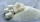2 teaspoons (24 g) of sugar are dissolved in a cup with 500 g of tea. Calculate the weight fraction of sugar in the tea.
• When 5When 5 is subtracted from both my numerator and denominator I become 3/4. What fraction i am?
• Athletics teamAll athletes in the athletics team from Ostrava can start in four, five, six and seven stages, and no one will be present. How many average athletes are in one athletic group if there are a total of twelve groups in the section? Consider the smallest numb
• TyronTyron attended his online class 2 hours a day. He already attend 1 3/4 hours, how many more hours will Tyron attend his class?
• Denissa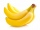Denissa has 18 9/10 kilos of bananas to sell if she was able to sell 15 ⅗. How many kilos of bananas were left?
• 1000 billA hat costs Php 75.25 and a discounted T-shirt costs Php 750.55. How much change will you receive if you pay for both items with a Php 1 000 bill?
• AlexandraAlexandra made a rectangular quilt the measured 3 1/4. 2 3/4 feet in width. What is the area of the quilt in square feet? Write an equation to solve.
• Evaluate 18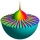Evaluate the expression (-4-7i)-(-6-9i) and write the result in the form a+bi (Real + i* Imaginary).
• Lowest terms 2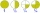The expression 4/12 can be expressed in its lowest term as 1/3. What is 3/15 expressed in its lowest term?
• Frame image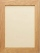Peter wants to put a picture in a frame. The picture is 7 3/5 cm wide. To fit in the picture frame cannot be more than 7 3/10 cm wide. How much should the image be trimmed?
• A rectangle 7A rectangle that has been split into 24 parts, set in 4 rows of 6 parts each. The parts in the top 3 rows have been shaded blue. The parts in the left 5 columns have been shaded purple. The first 5 parts in the top 3 rows are striped to show they are shad
• ShopperEva spent 1/4 in one store and 1/3 in another. What fraction is left?
• Three integersThree consecutive integers add up to 51. what are these integers?
• Original numberJoe divided a number by 2 1/4 to get 2/3. What was the original number?
• Cis notation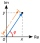Evaluate multiplication of two complex numbers in cis notation: (6 cis 120°)(4 cis 30°) Write result in cis and Re-Im notation.
• The sum 16The sum of A and B is 36. The difference between A & B is 8. find the larger number of A.
• The product 2The product of two functions is 10. If one of them is 2 1/3, find the other one.
• Multiplicative inverse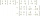Explain why the reciprocal of - 1 1/17 is the multiplicative inverse of - 17/18.
• Denominator 2Denominator of a fraction is 5 and numerator is 7. Write the fraction .

Do you have an exciting math question or word problem that you can't solve? Ask a question or post a math problem, and we can try to solve it.

We will send a solution to your e-mail address. Solved examples are also published here. Please enter the e-mail correctly and check whether you don't have a full mailbox.

Please do not submit problems from current active competitions such as Mathematical Olympiad, correspondence seminars etc...